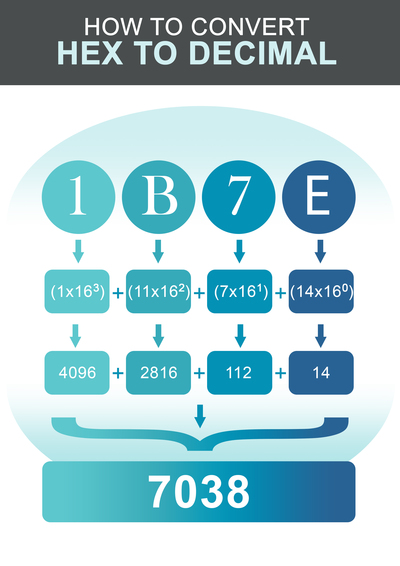Enter Hex Number:

## How to convert Hex to Decimal:Let's take 1B7E as a hexadecimal number and convert it into a decimal by going through the following steps:

Step 1: Mark the index to each digit in the hexadecimal number. The index simply is the position of the digit within the number counting from right to left.

\begin{array}{|c|c|c|c|c|c|c|} \hline \text{Hexadecimal } & \text{1} & \text{B} & \text{7} & \text{E} \\ \hline \text{Index } & \text{3} & \text{2} & \text{1} & \text{0} \\ \hline \end{array}

Step 2: Replace the digits with decimal equivalent values according to the given mapping:

\begin{array}{|c|c|c|c|c|c|c|} \hline \text{Hex code} & \text{A} & \text{B} & \text{C} & \text{D} & \text{E} & \text{F} \\ \hline \text{Dec Equiv.} & \text{10} & \text{11} & \text{12} & \text{13} & \text{14} & \text{15} \\ \hline \end{array}

For the given example the result can be written down like:

\begin{array}{|c|c|c|c|c|c|c|} \hline \text{Hexadecimal value in Decimal } & \text{1} & \text{11} & \text{7} & \text{14} \\ \hline \text{Index } & \text{3} & \text{2} & \text{1} & \text{0} \\ \hline \end{array}

Step 3: Now multiply each digit of the hexadecimal number with 16 raised to the power of their respective index to get the place value in decimal.

Convert the position of E: $$E \Rightarrow 14 \times 16^0 = 14$$
Convert the position of 7: $$7 \Rightarrow 7 \times 16^1 = 112$$
Convert the position of B: $$B \Rightarrow 11 \times 16^2 = 2816$$
Convert the position of 1: $$1 \Rightarrow 1 \times 16^3 = 4096$$

Step 4: Now add all the place values to get the decimal equivalent.

$$DEC = 4096 + 2816 + 112 + 14 = 7038$$

## The Hexadecimal and Decimal Number System in Comparison:

A Number system is an ordered set of specific symbols describing quantities; You might have heard of binary, decimal & hexadecimal number systems already.

## The Radix of a Number system

It is possible to represent any quantity in all number systems; The only difference between these number systems is the radix or the number of digits. The total number of distinct digits in a number system is known as the radix or the base of that number system.

## The Decimal Number System:

The decimal number system is the number system with radix (base) equal to 10. In any number system, there are two things: Face value and place value. Let's consider a random number like 245. We can write this number in the weighted form as:

$$245 = (2 \times 100) + (4 \times 10) + (5 \times 1)$$

In the above example, we multiply the face value 2 by the weight of its place, which is 100 first, and repeat the procedure for all other positions.

## The Hexadecimal Number System:

As the name suggests, this number system uses the base 16 system. In this number system, we have 16 distinct digits, which are 0, 1, 2, 3, 4, 5, 6, 7, 8, 9, A, B, C, D, E, and F. This number system is preferred for most of the computer storage and programming because it is the perfect fit between decimal and binary number systems.

## Why are some number systems more common than others?

A common question can arise: If we can build a number system on any base, why are we using binary, decimal, and hexadecimal the most, and why not any other number system?

The reasons are of both practical and historical nature: We can see that the decimal number system has the base 10, which is precisely the number of our fingers. This fact explains why the decimal number system has been so popular for such a long time.

The popularity of the binary system has suddenly increased with the age of computers that can operate on binary digits only; The disadvantage of the binary system is the length of binary numbers since the base only consists of two numbers.

The hexadecimal system is the perfect link between the binary and the decimal system: The minimum bits in the binary system required to denote the decimal number 10 is 4:

$$1010$$

However, with 4 bits it is possible to denote 16 different symbols or digits: The binary number 1111 corresponds to 16 in the decimal system. And this is how hexadecimal came into the picture. When using 4 bits to denote 10 digits only, we the other six digits. Using hexadecimal numbers, we can represent bigger numbers with fewer bits, and there is no waste of memory.